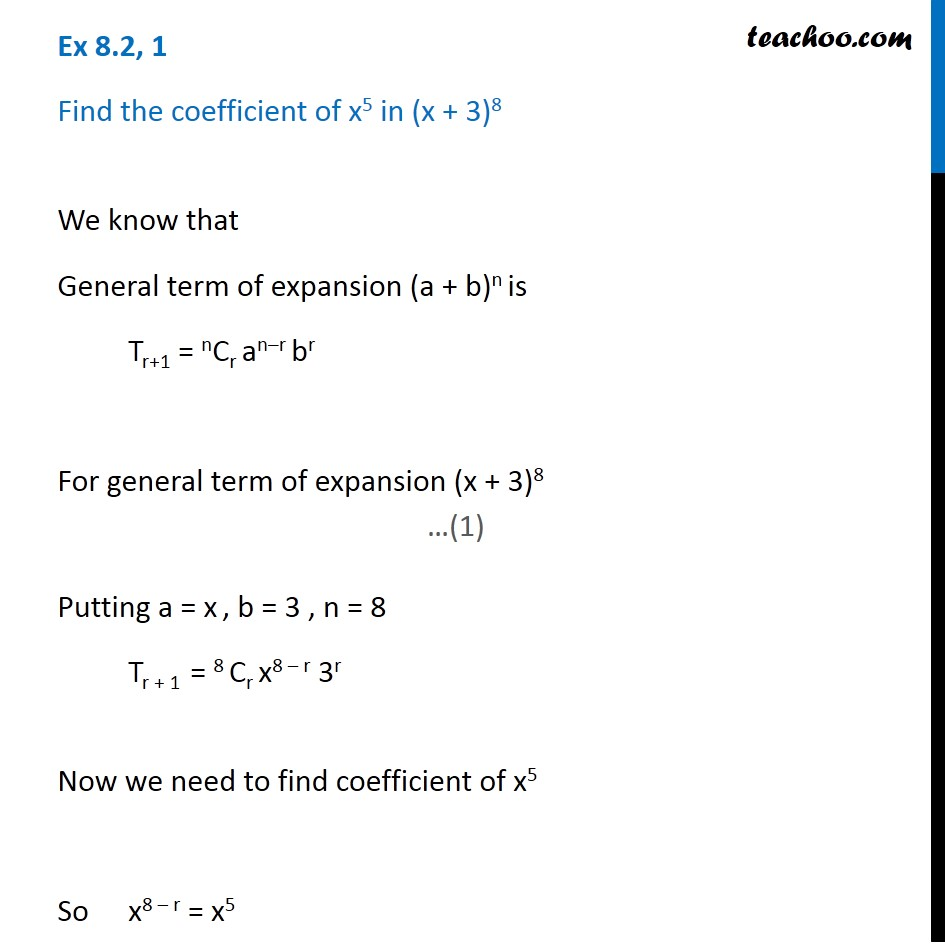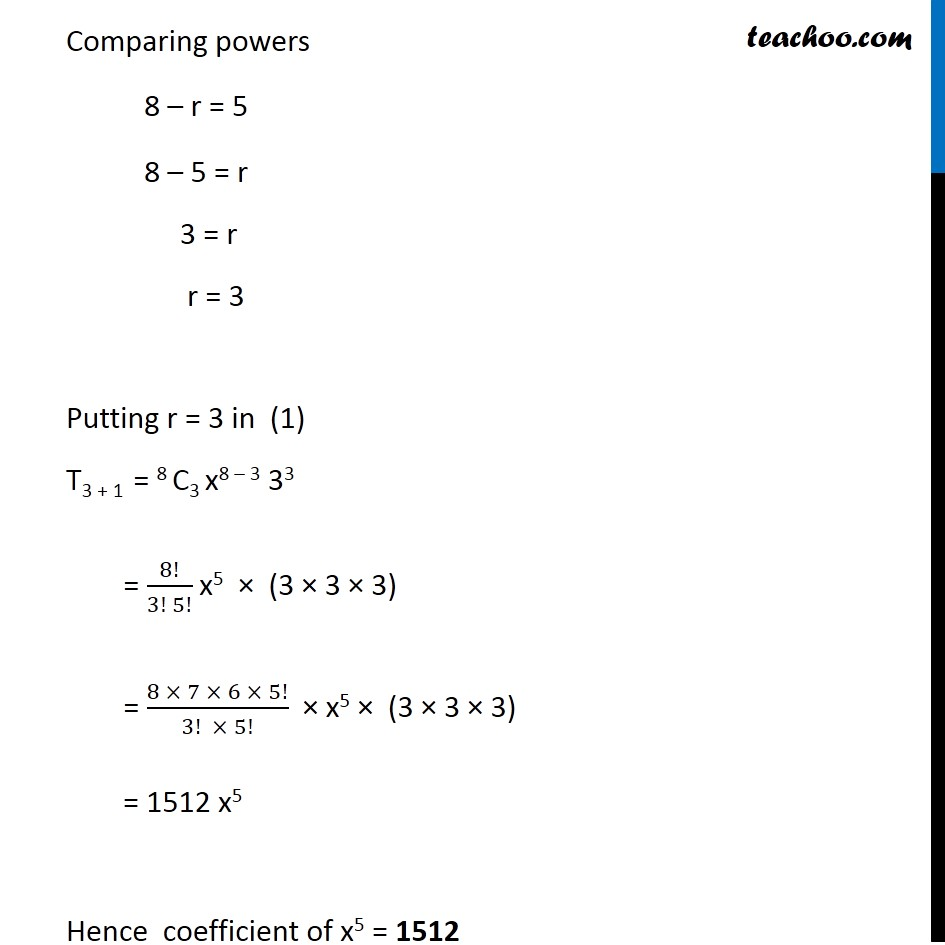General Term of Binomial Theorem

Chapter 7 Class 11 Binomial Theorem
Serial order wiseThis video is only available for Teachoo black users

Learn in your speed, with individual attention - Teachoo Maths 1-on-1 Class

### Transcript

Question 1 - Introduction Find the coefficient of x5 in (x + 3)8 In 18x5 Coefficient of x5 = 18 Question 1 Find the coefficient of x5 in (x + 3)8 We know that General term of expansion (a + b)n is Tr+1 = nCr an–r br For general term of expansion (x + 3)8 Putting a = x , b = 3 , n = 8 Tr + 1 = 8 Cr x8 – r 3r Now we need to find coefficient of x5 So x8 – r = x5 Comparing powers 8 – r = 5 8 – 5 = r 3 = r r = 3 Putting r = 3 in (1) T3 + 1 = 8 C3 x8 – 3 33 = 8!/(3! 5!) x5 × (3 × 3 × 3) = (8 × 7 × 6 × 5!)/(3! × 5!) × x5 × (3 × 3 × 3) = 1512 x5 Hence coefficient of x5 = 1512